# Four different dynamical systems

This multimedia post features four different dynamical systems to illustrate some properties of that relevant to my research: we’ll talk about the difference between “integrable” and “chaotic” dynamical systems, and the difference between “continuous-time” and “discrete-time” dynamical systems.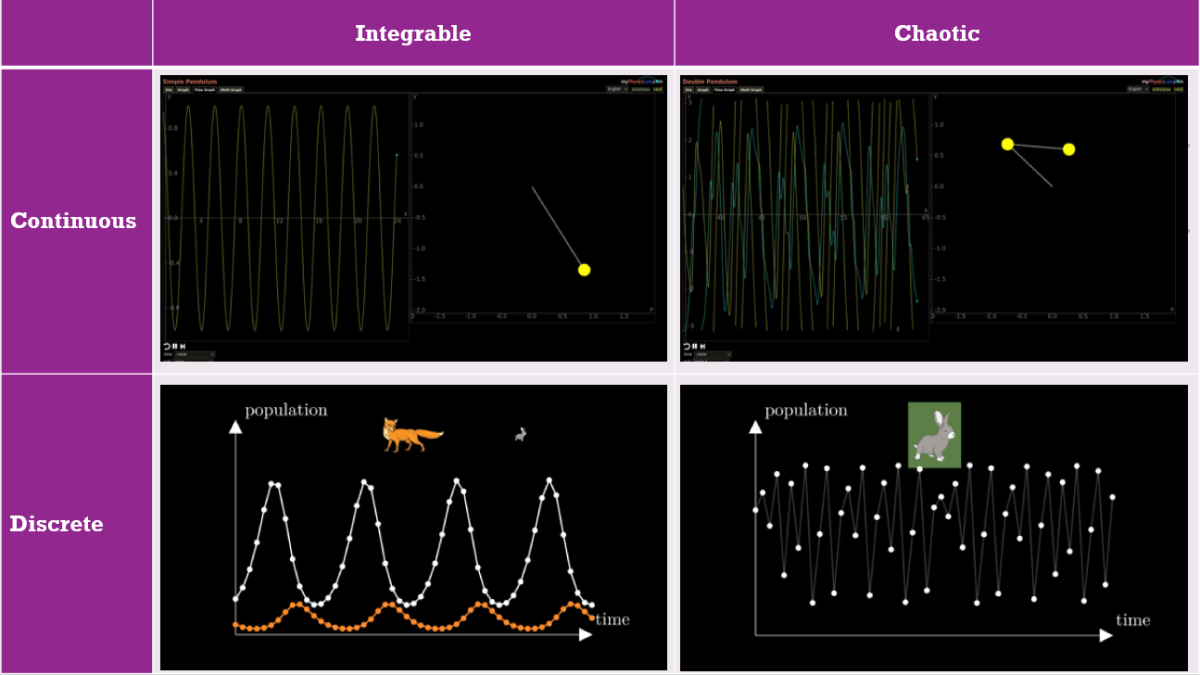## Introduction

This is an extended write-up of a five-minute talk I gave to an interdisciplinary audience at Loughborough University’s 2022 Research Conference. It features four different :dynamical systems to illustrate some properties of dynamical systems relevant to my research. In particular, we’ll talk about the difference between “integrable” and “chaotic” dynamical systems, and the difference between “continuous-time” and “discrete-time” dynamical systems. So we’ll want to fill up this grid with examples: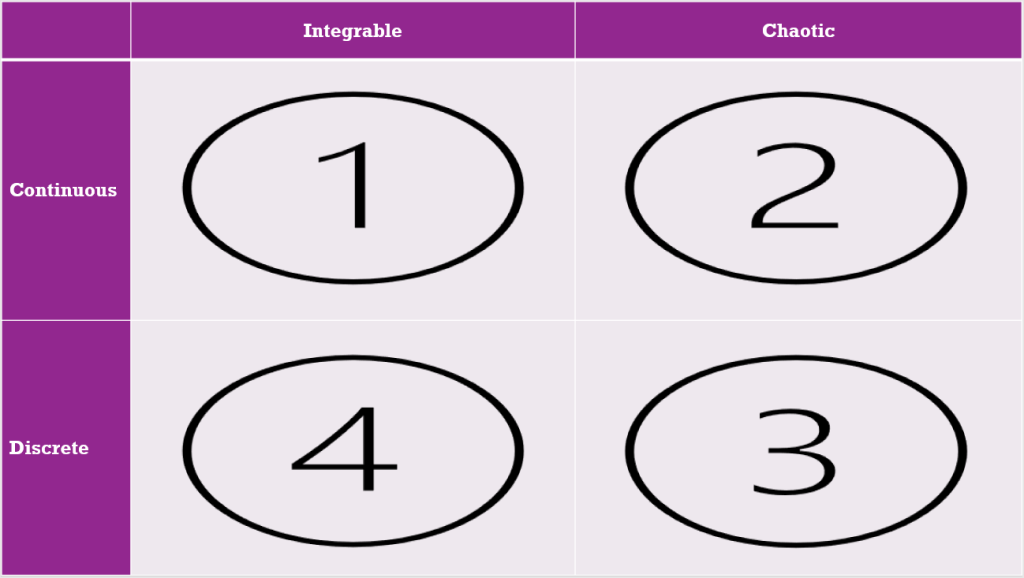## Continuous-time and integrable

First, let’s consider a :simple pendulum. (Yes, that is the technical term.) This is an object suspended from a string or from a rod that can freely rotate around a hinge. If we give the pendulum a little push, then (ignoring friction) it will keep swinging back and forth again and again.

The graph traced out on the left shows the angle of deflection as a function of time. It is very predictable, and we could write down a formula that precisely describes this graph. The pendulum is an example of an integrable system. “Integrable” is an archaic word for “solvable”: integrable systems are dynamical systems for which we could write down a formula that exactly describes its solution (its state as a function of time). The behaviour of an integrable system is ordered and predictable.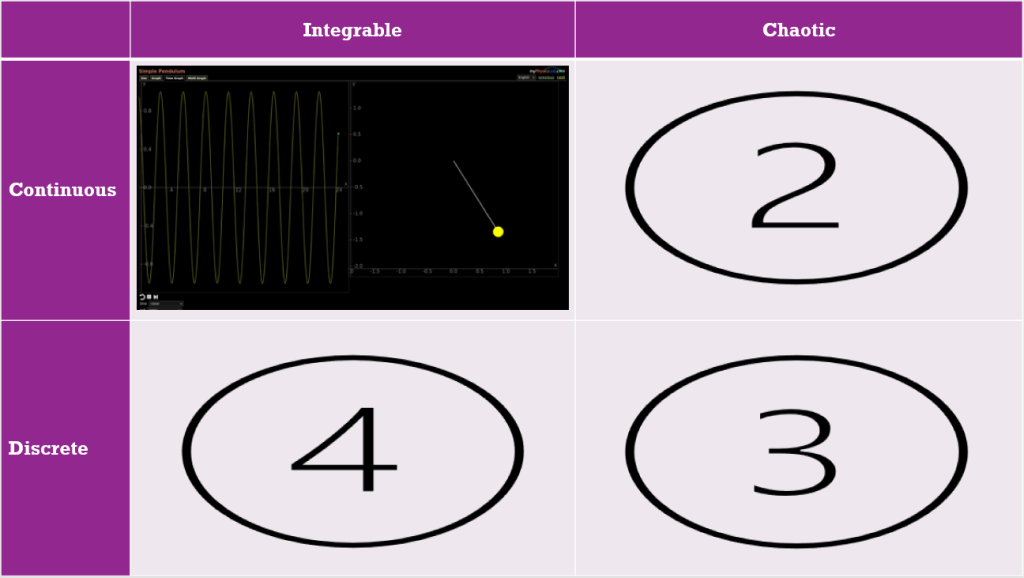## Continuous-time and chaotic

If we attach another pendulum to a simple pendulum, we get a double pendulum. (Yes, that is the technical term.) Let’s give it a good shove and see what happens:

There are now two graphs on the left, representing the angles of both pieces of the pendulum. However, the main difference with the simple pendulum is that these graphs don’t show any repetition or pattern. In fact it is impossible to write down a formula that produces these graphs exactly. Looking at the motion of the system, it is very hard to predict what position it we be in a few seconds into the future. Furthermore, the evolution of the system is :very sensitive to changes in its initial state. These are the properties of a :chaotic system.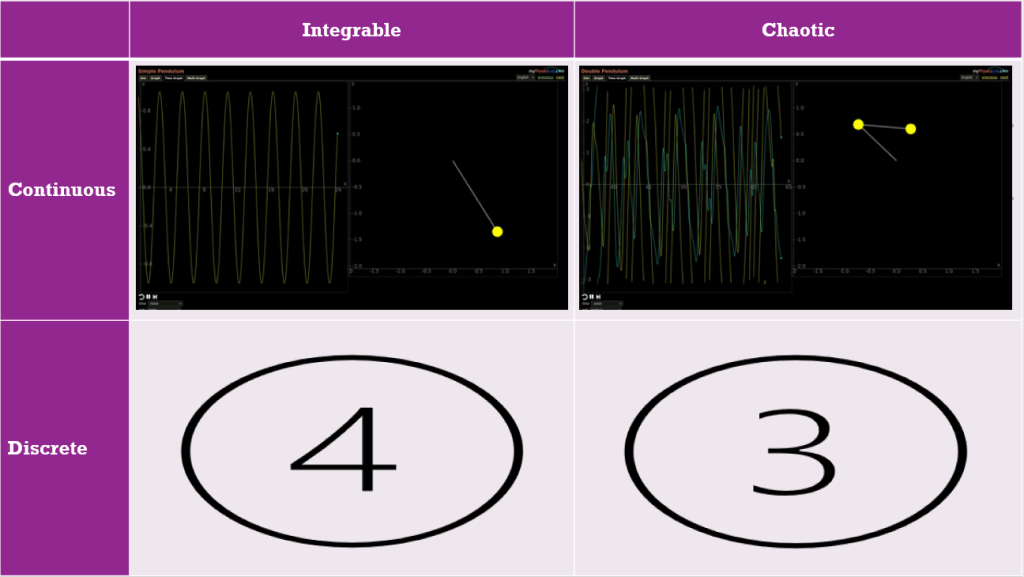You could think of integrable systems and chaotic systems as opposite ends of a wide spectrum representing how dynamical systems can behave.

## Discrete-time and chaotic

So far, our systems have evolved :continuously in time: they don’t jump instantaneously from a certain state to a completely different one. There are also systems where it doesn’t make sense to keep track of the configuration at every instant. Consider for example a population of animals that breed once per year, at a fixed time of year. To keep track of the population we don’t need to count them every minute of every day. We can just do one count per year at the end of the breeding season. We can then use this count to predict what the population in the next year will be. Such a model is a discrete-time dynamical system: it takes steps in time of a fixed size.

The following model tracks a population of :rabbits living in a certain habitat with limited resources. If the population is small they all have plenty to eat and will produce large litters. If the population is large they compete for resources and will have smaller litters or even die of starvation. We see that the population fluctuates wildly over the years:

These oscillations do not follow a fixed pattern: sometimes the population grows two years in a row, sometimes its goes up and down in alternating years. This makes it very difficult to predict what will happen a few years in the future. So this discrete-time dynamical system is chaotic.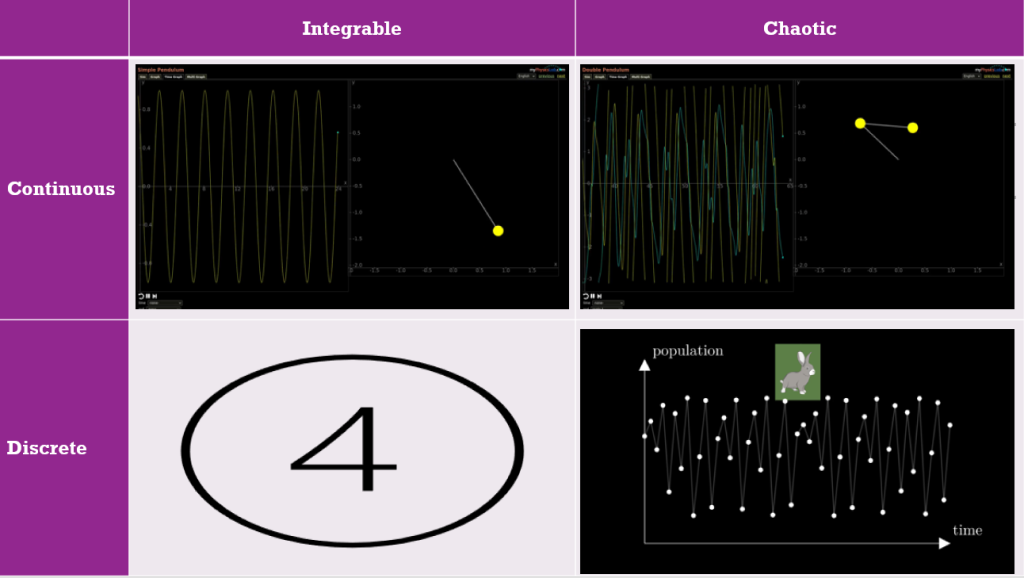## Discrete-time and integrable

In the next :model the rabbits always have plenty to eat, but they are being hunted by foxes.

If there are only a few foxes, this does not impact the rabbit population very much and it grows quickly. But then, with many rabbits to eat, the foxes do quite well for themselves. After a few years there are so many foxes that a large proportion of the rabbits gets eaten. The rabbit population declines sharply, leaving the large fox population struggling to find food. This causes the fox population to decline, and the cycle starts over.

In this case the oscillations follow a fixed pattern: the population sizes are predictable and we could theoretically write a formula for the graphs they trace. This discrete-time dynamical system is integrable.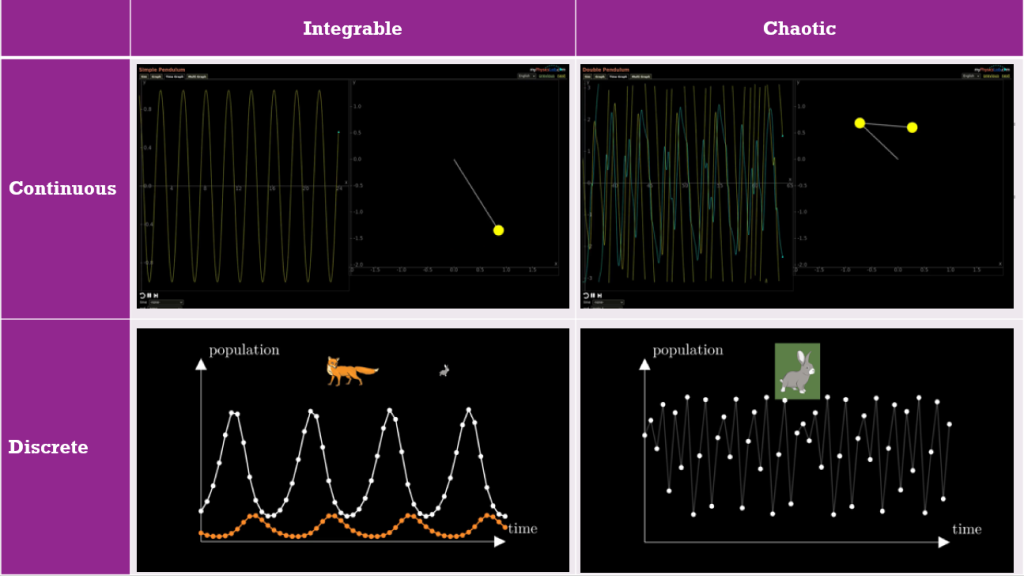## Integrable systems are the exception

The simple pendulum is quite, well, simple. Also the predator-prey model describing our rabbits and foxes is much simpler than any realistic ecological model. There are more complicated integrable systems, but they are relatively rare. If a dynamical system is sufficiently complicated, it is usually chaotic. Integrable systems are the exception. Their nice behaviour and predictabillity is due to some :hidden mathematical structure they possess. This structure can take many different forms. There isn’t one type of hidden structure that explains all integrable systems. Studying all these structures and the relations between them is an active are of research.

Many discrete integrable systems have continuous counterparts, and vice versa. Making the connections between discrete and continuous integrable systems precise often sheds light on their hidden structures. :This approach to studying integrable systems is one of the topics of my research.

## :Credits

The first two videos are edited screengrabs from https://www.myphysicslab.com.

The last two videos are my own work [source code] made using Manim and public domain images.

Expandable notes are implemented using :Nutshell.

## :x Nutshell

These expandable notes are made using :Nutshell ← Click on the : to expand nested nutshells.

## :x D S

The mathematical model of anything that moves or changes is called a dynamical system.

## :x T T

Yes, that is the technical term.

## :x D PThree double pendulums with near identical initial conditions diverge over time displaying the chaotic nature of the system. [From Wikipedia]

## :x C C

In mathematics, a function is “continuous” if small changes in input lead to small changes in output. In the present context we consider the state of a dynamical system as a function of time, so “continuous” means that if we move forward a very small amount of time, then the change in the system’s state will be correspondingly small.

## :x B L B

These rabbits don’t breed like bunnies! They can have large litters, yes, but they only reproduce once per year.

## :x L V

This is a discrete-time version of the :Lotka-Volterra model.

## :x D S I

For readers with a maths background who want to learn more about discrete integrable systems, I recommend the book Discrete Systems and Integrability by J. Hietarinta, N. Joshi and F. W. Nijhoff.

## :x L M S

Readers with a maths background who want find out what some of these structures are, could watch for example the 2020 LMS lecture series Introduction to Integrability.

If you’re after more visual representations on integrable systems, check out What is… an integrable system? where I give a different introduction to integrable systems, using waves as examples.